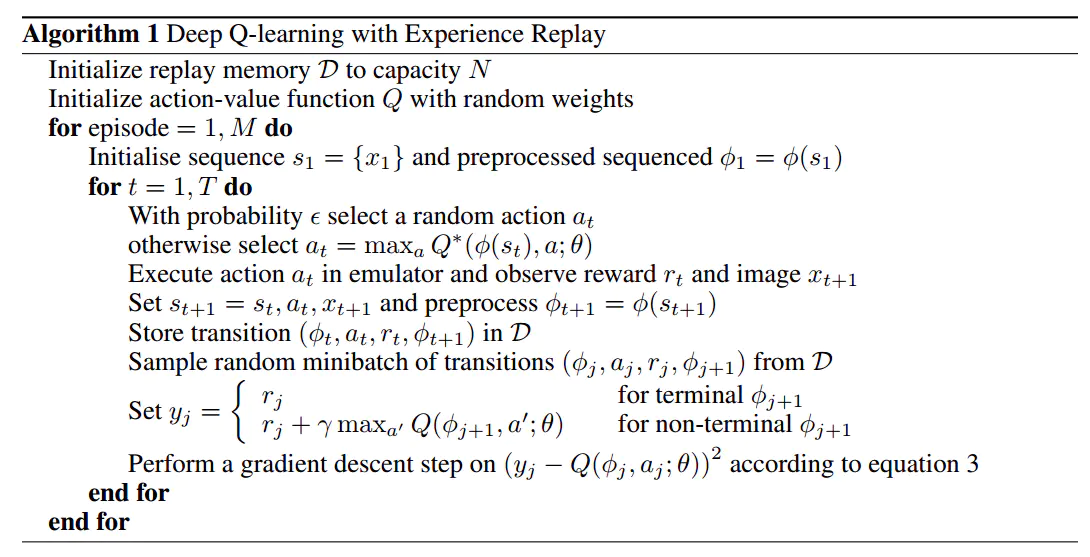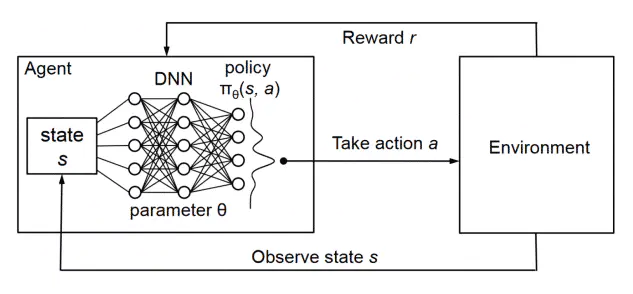# DQN简介

Q-learning算法很早就有了，但是其与深度学习的结合是在2013年的DeepMind发布的《Playing Atari with Deep Reinforcement Learning》论文中才实现的。这篇论文创造性的将RL与DL实现了融合，提出了存储记忆（Experience Replay）机制和Fixed-Q-Target，实现了一部分Atari游戏操控，甚至超过了人类水平。# DQN代码实现

## OpenAI gym

openAI开发的python第三方库gym，是一个现阶段主流的强化学习入门的环境。可以为强化学习算法提供许多游戏或者控制场景的接口，让开发者更多关注在算法的研究上而不是环境的设计。``````#gym库的基本使用操作如下
import gym
env = gym.make("CartPole-v1")      #调用该环境
observation = env.reset()		   #重置环境
for _ in range(1000):
env.render()                     #打开环境窗口
action = env.action_space.sample() # 从行为空间随机挑选动作
observation, reward, done, info = env.step(action)   #执行一步action之后的返回值

if done:
observation = env.reset()
env.close()                         #关闭环境
``````

``````import torch                                    # 导入torch
import torch.nn as nn                           # 导入torch.nn
import torch.nn.functional as F                 # 导入torch.nn.functional
import numpy as np                              # 导入numpy
import gym                                      # 导入gym

# 超参数
BATCH_SIZE = 32                                 # 样本数量
LR = 0.01                                       # 学习率
EPSILON = 0.9                                   # greedy policy
GAMMA = 0.9                                     # reward discount
TARGET_REPLACE_ITER = 100                       # 目标网络更新频率
MEMORY_CAPACITY = 2000                          # 记忆库容量
env = gym.make('CartPole-v0').unwrapped         # 使用gym库中的环境：CartPole，且打开封装
N_ACTIONS = env.action_space.n                  # 杆子动作个数 (2个)
N_STATES = env.observation_space.shape       # 杆子状态个数 (4个)
``````

``````# 定义Net类 (定义网络)
class Net(nn.Module):
def __init__(self):                                                         # 定义Net的一系列属性
# nn.Module的子类函数必须在构造函数中执行父类的构造函数
super(Net, self).__init__()                                             # 等价与nn.Module.__init__()

self.fc1 = nn.Linear(N_STATES, 50)                                      # 设置第一个全连接层(输入层到隐藏层): 状态数个神经元到50个神经元
self.fc1.weight.data.normal_(0, 0.1)                                    # 权重初始化 (均值为0，方差为0.1的正态分布)
self.out = nn.Linear(50, N_ACTIONS)                                     # 设置第二个全连接层(隐藏层到输出层): 50个神经元到动作数个神经元
self.out.weight.data.normal_(0, 0.1)                                    # 权重初始化 (均值为0，方差为0.1的正态分布)

def forward(self, x):                                                       # 定义forward函数 (x为状态)
x = F.relu(self.fc1(x))                                                 # 连接输入层到隐藏层，且使用激励函数ReLU来处理经过隐藏层后的值
actions_value = self.out(x)                                             # 连接隐藏层到输出层，获得最终的输出值 (即动作值)
return actions_value                                                    # 返回动作值

``````

``````# 定义DQN类 (定义两个网络)
class DQN(object):
def __init__(self):                                                         # 定义DQN的一系列属性
self.eval_net, self.target_net = Net(), Net()                           # 利用Net创建两个神经网络: 评估网络和目标网络
self.learn_step_counter = 0                                             # for target updating
self.memory_counter = 0                                                 # for storing memory
self.memory = np.zeros((MEMORY_CAPACITY, N_STATES * 2 + 2))             # 初始化记忆库，一行代表一个transition
self.loss_func = nn.MSELoss()                                           # 使用均方损失函数 (loss(xi, yi)=(xi-yi)^2)

def choose_action(self, x):                                                 # 定义动作选择函数 (x为状态)
x = torch.unsqueeze(torch.FloatTensor(x), 0)                            # 将x转换成32-bit floating point形式，并在dim=0增加维数为1的维度
if np.random.uniform() < EPSILON:                                       # 生成一个在[0, 1)内的随机数，如果小于EPSILON，选择最优动作
actions_value = self.eval_net.forward(x)                            # 通过对评估网络输入状态x，前向传播获得动作值
action = torch.max(actions_value, 1).data.numpy()                # 输出每一行最大值的索引，并转化为numpy ndarray形式
action = action                                                  # 输出action的第一个数
else:                                                                   # 随机选择动作
action = np.random.randint(0, N_ACTIONS)                            # 这里action随机等于0或1 (N_ACTIONS = 2)
return action                                                           # 返回选择的动作 (0或1)

def store_transition(self, s, a, r, s_):                                    # 定义记忆存储函数 (这里输入为一个transition)
transition = np.hstack((s, [a, r], s_))                                 # 在水平方向上拼接数组
# 如果记忆库满了，便覆盖旧的数据
index = self.memory_counter % MEMORY_CAPACITY                           # 获取transition要置入的行数
self.memory[index, :] = transition                                      # 置入transition
self.memory_counter += 1                                                # memory_counter自加1

def learn(self):                                                            # 定义学习函数(记忆库已满后便开始学习)
# 目标网络参数更新
if self.learn_step_counter % TARGET_REPLACE_ITER == 0:                  # 一开始触发，然后每100步触发
self.learn_step_counter += 1                                            # 学习步数自加1

# 抽取记忆库中的批数据
sample_index = np.random.choice(MEMORY_CAPACITY, BATCH_SIZE)            # 在[0, 2000)内随机抽取32个数，可能会重复
b_memory = self.memory[sample_index, :]                                 # 抽取32个索引对应的32个transition，存入b_memory
b_s = torch.FloatTensor(b_memory[:, :N_STATES])
# 将32个s抽出，转为32-bit floating point形式，并存储到b_s中，b_s为32行4列
b_a = torch.LongTensor(b_memory[:, N_STATES:N_STATES+1].astype(int))
# 将32个a抽出，转为64-bit integer (signed)形式，并存储到b_a中 (之所以为LongTensor类型，是为了方便后面torch.gather的使用)，b_a为32行1列
b_r = torch.FloatTensor(b_memory[:, N_STATES+1:N_STATES+2])
# 将32个r抽出，转为32-bit floating point形式，并存储到b_s中，b_r为32行1列
b_s_ = torch.FloatTensor(b_memory[:, -N_STATES:])
# 将32个s_抽出，转为32-bit floating point形式，并存储到b_s中，b_s_为32行4列

# 获取32个transition的评估值和目标值，并利用损失函数和优化器进行评估网络参数更新
q_eval = self.eval_net(b_s).gather(1, b_a)
# eval_net(b_s)通过评估网络输出32行每个b_s对应的一系列动作值，然后.gather(1, b_a)代表对每行对应索引b_a的Q值提取进行聚合
q_next = self.target_net(b_s_).detach()
# q_next不进行反向传递误差，所以detach；q_next表示通过目标网络输出32行每个b_s_对应的一系列动作值
q_target = b_r + GAMMA * q_next.max(1).view(BATCH_SIZE, 1)
# q_next.max(1)表示只返回每一行的最大值，不返回索引(长度为32的一维张量)；.view()表示把前面所得到的一维张量变成(BATCH_SIZE, 1)的形状；最终通过公式得到目标值
loss = self.loss_func(q_eval, q_target)
# 输入32个评估值和32个目标值，使用均方损失函数
loss.backward()                                                 # 误差反向传播, 计算参数更新值
self.optimizer.step()                                           # 更新评估网络的所有参数
``````

``````dqn = DQN()                                                             # 令dqn=DQN类

for i in range(400):                                                    # 400个episode循环
print('<<<<<<<<<Episode: %s' % i)
s = env.reset()                                                     # 重置环境
episode_reward_sum = 0                                              # 初始化该循环对应的episode的总奖励

while True:                                                         # 开始一个episode (每一个循环代表一步)
env.render()                                                    # 显示实验动画
a = dqn.choose_action(s)                                        # 输入该步对应的状态s，选择动作
s_, r, done, info = env.step(a)                                 # 执行动作，获得反馈

# 修改奖励 (不修改也可以，修改奖励只是为了更快地得到训练好的摆杆)
x, x_dot, theta, theta_dot = s_
r1 = (env.x_threshold - abs(x)) / env.x_threshold - 0.8
new_r = r1 + r2

dqn.store_transition(s, a, new_r, s_)                 # 存储样本
episode_reward_sum += new_r                           # 逐步加上一个episode内每个step的reward

s = s_                                                # 更新状态

if dqn.memory_counter > MEMORY_CAPACITY:              # 如果累计的transition数量超过了记忆库的固定容量2000
# 开始学习 (抽取记忆，即32个transition，并对评估网络参数进行更新，并在开始学习后每隔100次将评估网络的参数赋给目标网络)
dqn.learn()

if done:       # 如果done为True
# round()方法返回episode_reward_sum的小数点四舍五入到2个数字
print('episode%s---reward_sum: %s' % (i, round(episode_reward_sum, 2)))
break                                             # 该episode结束
env.close()
``````

# 总结

DQN是深度学习和强化学习结合的一个例子，在游戏操控领域大放异彩，其本质思想仍然是Q-learning的时序差分算法和贪婪策略思想。在借助了神经网络的作用下，实现了价值函数近似的功能，并且利用经验回放机制和双神经网络架构，保证了算法的稳定性，降低了过拟合的概率。本文只是对于DQN入门级别的介绍。后续在学习过程中还会不断补充更加进一步地学习强化学习。